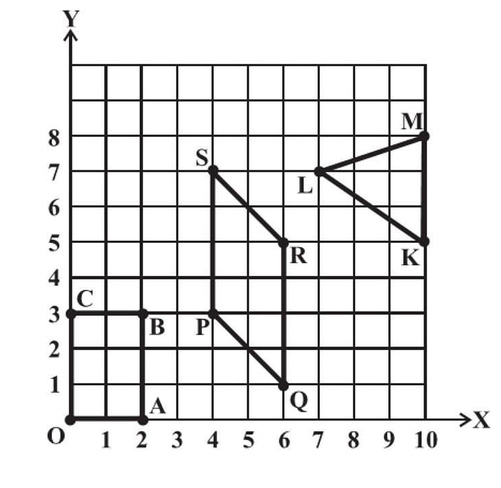# Ex.15.2 Q3 Introduction to Graphs Solutions - NCERT Maths Class 8

## Question

Write the coordinates of the vertices of each of these adjoining figures.Video Solution
Introduction To Graphs
Ex 15.2 | Question 3

## Text Solution

Reasoning:

$$x$$-axis and $$y$$-axis are represented as a number line starting from ($$0,0$$). Locate the numerical value of the given points.

Steps:

The coordinates of the vertices in the given figure are as follows.

\begin{align}&{{O(0,0), A(2,0), B(2,3),C(0,3)}}\\&{{P(4,3), Q(6,1), R(6,5),S(4,7)}}\\&{{K(10,5), L(7,7),M (10,8)}}\end{align}

Learn from the best math teachers and top your exams

• Live one on one classroom and doubt clearing
• Practice worksheets in and after class for conceptual clarity
• Personalized curriculum to keep up with school